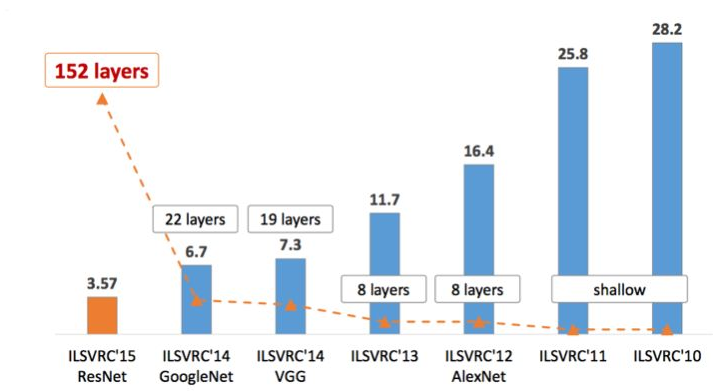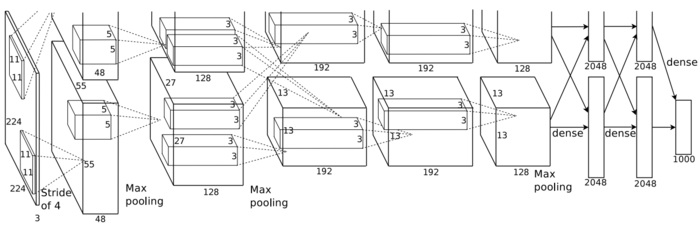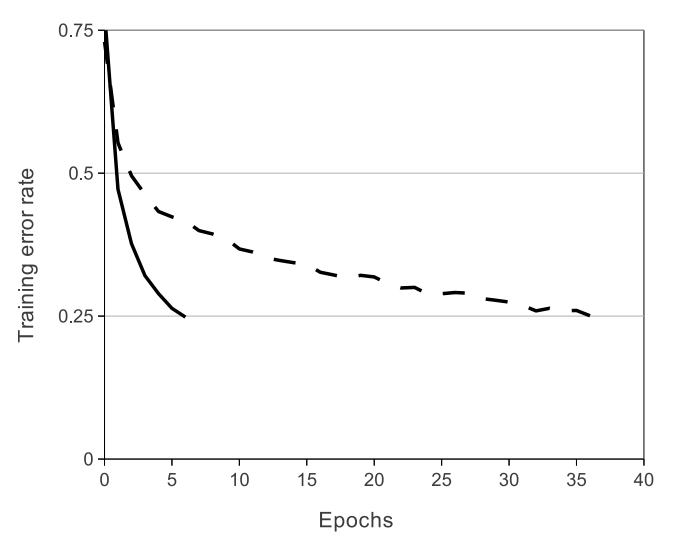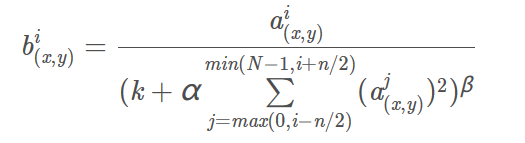• 更深的网络
• ReLU激活函数
• Dropout
• LRN
• 数据增强

# 网络结构¶

AlexNet的网络结构如下图所示：• 数据输入，尺寸为$[224,224,3]$
• 第一个卷积层conv1的卷积核尺寸为$11\times 11$，滑动步长为$4$，卷积核数目为$48$。卷积后得到的输出矩阵维度为$[48,55,55]$。值得注意的是如果直接按照卷积的定义来计算的话，那么输出特征的长宽应该是$(224-11)/4+1$，这个值并不是$55$，因此这里的值是将原图做了padding之后再进行卷积得到的，具体来说就是将原图padding到$227\times 227$，这样再计算就是$(227-11)/4+1=55$了。所以这一层的输出特征图维度就是$[48, 55, 55]$
• 第一个卷积层后面接一个激活函数ReLU层。处理后的特征图维度仍为$[48,55,55]$
• 将经过激活函数处理的特征图通过一个LRN层。输出维度仍为$[48,55,55]$
• 接下来是第一个池化层。使用的是Max Pooling池化操作，池化操作的池化核大小为$3\times 3$，步长为$2$，所以池化后的特征图尺寸为$(55-3)/2+1=27$。因此输出特征图维度为$[48,27,27]$
• 第二个卷积层conv2输入为上一层的特征图，卷积核的个数为$128$个，注意这是第一个分支，第二个分支每层的结构和第一层完全一致，也就是说现在在显卡显存够的情况下可以直接设置第二个卷积层的通道数为$128\times 2=256$。卷积核的大小为$5\times 5$$pad=2$$stride=1$，同样也经过ReLU，LRN，然后再经过MaxPooling层，其中pool_size=（3,3）,stride=2。最后输出特征图维度为$[13,13,128]$
• 第三个卷积层conv3输入为上一层的输出特征图，卷积核个数为$192$，卷积核尺寸为$3\times 3$$pad=1$，没有做LRN和Max Pooling。
• 第四个卷积层输入为第三个卷积层的输出，卷积核个数为$192$，卷积核尺寸为$3\times 3$$pad=1$，没有做LRN和Max Pooling。
• 第五个卷积层为第四个卷积层的输出，卷积核个数为$128$，卷积核尺寸为$3\times 3$$pad=1$。然后接一个MaxPooling层，其中pool_size=（3,3）,stride=2
• 第六，七，八层为全连接层，每一层的神经元个数是$2048$，最终输出softmax为$1000$，即ImageNet分类比赛中目标的种类数。全连接层中使用了ReLU和Dropout。

# ReLU¶

AlexNet之前神经网络一般使用tanh或者sigmoid作为激活函数，sigmoid激活函数的表达式为：

$f(x)=\frac{1}{1+e^{-x}}$

tanh激活函数的表达式为：

$tanh(x)=\frac{sinhx}{coshx}=\frac{e^x-e^{-x}}{e^x+e^{-x}}$

$ReLU(x)=max(0,x)$# Local Response Normalization(局部响应归一化)¶

AlexNet中认为ReLU激活函数的值域没有一个固定的区间（sigmoid激活函数值域为(0,1)），所以需要对ReLU得到的结果进行归一化，这一点有点像之后的BN，都是改变中间特征图权重分布加速收敛。即论文提出的Local Response Normalization。局部响应归一化的计算公式如下：• i ：代表下标，即要计算的像素值的下标，从0开始开始算起。
• j ：代表平方累加索引，即从j~i的像素值平方求和。
• x,y：代表像素中的位置，公式中用不到。
• a：代表特征图里面i对应的像素值。
• N：每个特征图最内层向量的列数。
• k：超参数，由网络层中的blas指定。
• alpha：超参数，由网络层中的alpha指定。
• n/2：超参数，由网络层中的deepth_radius指定。
• beta：超参数，由网络层中的belta指定。

import tensorflow as tf

a = tf.constant([
[[1.0, 2.0, 3.0, 4.0],
[5.0, 6.0, 7.0, 8.0],
[8.0, 7.0, 6.0, 5.0],
[4.0, 3.0, 2.0, 1.0]],
[[4.0, 3.0, 2.0, 1.0],
[8.0, 7.0, 6.0, 5.0],
[1.0, 2.0, 3.0, 4.0],
[5.0, 6.0, 7.0, 8.0]]
])
#reshape 1批次  2x2x8的feature map
a = tf.reshape(a, [1, 2, 2, 8])

normal_a=tf.nn.lrn(a,2,0,1,1)
with tf.Session() as sess:
print("feature map:")
image = sess.run(a)
print (image)
print("normalized feature map:")
normal = sess.run(normal_a)
print (normal)


feature map:
[[[[ 1.  2.  3.  4.  5.  6.  7.  8.]
[ 8.  7.  6.  5.  4.  3.  2.  1.]]

[[ 4.  3.  2.  1.  8.  7.  6.  5.]
[ 1.  2.  3.  4.  5.  6.  7.  8.]]]]
normalized feature map:
[[[[ 0.07142857  0.06666667  0.05454545  0.04444445  0.03703704  0.03157895
0.04022989  0.05369128]
[ 0.05369128  0.04022989  0.03157895  0.03703704  0.04444445  0.05454545
0.06666667  0.07142857]]

[[ 0.13793103  0.10000001  0.0212766   0.00787402  0.05194805  0.04
0.03448276  0.04545454]
[ 0.07142857  0.06666667  0.05454545  0.04444445  0.03703704  0.03157895
0.04022989  0.05369128]]]]


# Dropout¶

Dropout原理类似于浅层学习算法的中集成算法，该方法通过让全连接层的神经元（该模型在前两个全连接层引入Dropout）以一定的概率失去活性（比如0.5）失活的神经元不再参与前向和反向传播，相当于约有一半的神经元不再起作用。在测试的时候，让所有神经元的输出乘0.5。当然在实际实现Dropout层的时候是在训练阶段所有Dropout保留下来的神经元的权值直接除以p，这样在测试过程中就不用额外操作了。Dropout的引用，有效缓解了模型的过拟合。关于为什么要除以p，以及Dropout实现细节由于比较复杂之后单独开一篇文章讲一讲。这个地方知道他可以降低过拟合的风险并且对准确率有提升就够了。

# 代码实现¶

# AlexNet
def AlexNet(self):
model = Sequential()
# input_shape = (64,64, self.config.channles)
input_shape = (self.config.normal_size, self.config.normal_size, self.config.channles)
return model


# 卷积神经网络学习路线往期文章¶